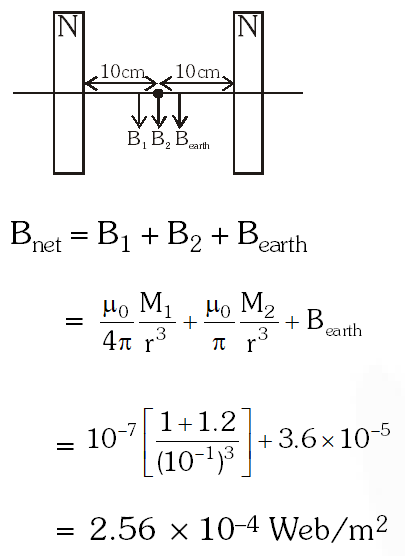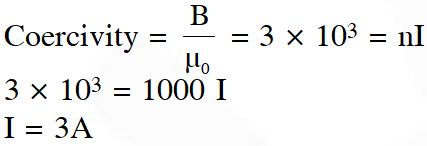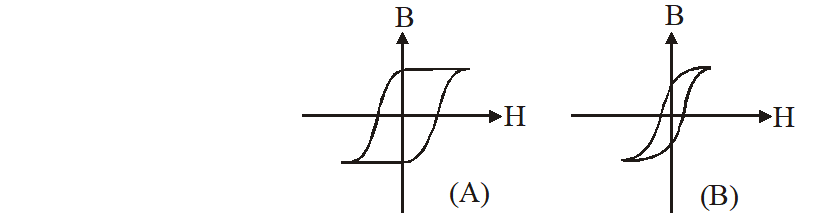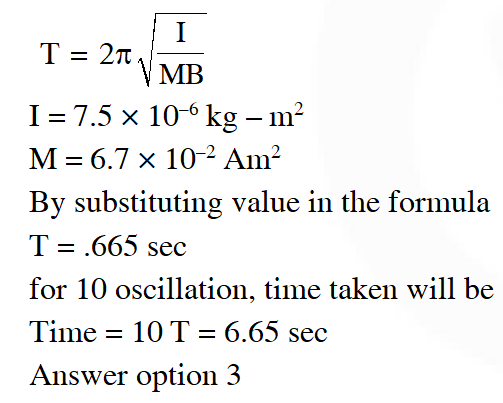Most Affordable JEE | NEET | 8,9,10 Preparation by Kota's Top IITian Doctor Faculties

# Magnetism - JEE Main Previous Year Questions with SolutionsJEE Main Previous Year Question of Physics with Solutions are available here. Practicing JEE Main Previous Year Papers Questions of Physics will help all the JEE aspirants in realizing the question pattern as well as help in analyzing their weak & strong areas. Get detailed Class 11th &12th Physics Notes to prepare for Boards as well as competitive exams like IIT JEE, NEET etc. eSaral helps the students in clearing and understanding each topic in a better way. eSaral is providing complete chapter-wise notes of Class 11th and 12th both for all subjects. Besides this, eSaral also offers NCERT Solutions, Previous year questions for JEE Main and Advance, Practice questions, Test Series for JEE Main, JEE Advanced and NEET, Important questions of Physics, Chemistry, Math, and Biology and many more. Download eSaral app for free study material and video tutorials. Simulator Previous Years AIEEE/JEE Mains Questions
Q. Due to the presence of the current $\mathrm{I}_{1}$ at the origin:- (1) The magnitude of the net force on the loop is given by $\frac{\mathrm{I}_{1} \mathrm{I}}{4 \pi} \mu_{0}\left[2(\mathrm{b}-\mathrm{a})+\frac{\pi}{3}(\mathrm{a}+\mathrm{b})\right]$ (2) The magnitude of the net force on the loop is given by $\frac{\mu_{0} \mathrm{I} \mathrm{I}_{1}}{24 \mathrm{ab}}(\mathrm{b}-\mathrm{a})$ (3) The forces on $\mathrm{AB}$ and $\mathrm{DC}$ are zero (4) The forces on $\mathrm{AD}$ and $\mathrm{BC}$ are zero [AIEEE - 2009]
Ans. (4) For AD and BC, $\overrightarrow{\mathrm{B}} \square \overrightarrow{\mathrm{dL}}$. Hence force on AD and BC is zero.
Q. Two short bar magnets of length 1 cm each have magnetic moments $1.20 \mathrm{Am}^{2}$ and $1.00 \mathrm{Am}^{2}$ respectively. They are placed on a horizontal table parallel to each other with their N poles pointing towards the South. They have a common magnetic equator and are separated by a distance of 20.0 cm. The value of the resultant horizontal magnetic induction at the midpoint O of the line joining their centres is close to :- (Horizontal component of earth's magnetic induction is $\left.3.6 \times 10^{-5} \mathrm{Wb} / \mathrm{m}^{2}\right)$ (1) $3.6 \times 10^{-5} \mathrm{Wb} / \mathrm{m}^{2}$ (2) $2.56 \times 10^{-4} \mathrm{Wb} / \mathrm{m}^{2}$ (3) $3.50 \times 10^{-4} \mathrm{Wb} / \mathrm{m}^{2}$ (4) $5.80 \times 10^{-4} \mathrm{Wb} / \mathrm{m}^{2}$ [JEE(Mains) - 2013]
Ans. (2)Q. The coercivity of a small magnet where the ferromagnet gets demagnetized is $3 \times 10^{3} \mathrm{A} \mathrm{m}^{-1}$. The current required to be passed in a solenoid of length 10 cm and number of turns 100, so that the magnet gets demagnetized when inside the solenoid, is : (1) 3A          (2) 6 A            (3) 30 mA             (4) 60 mA [JEE(Mains) - 2014]
Ans. (1)Q. Hysteresis loops for two magnetic materials A and B are given below :These materials are used to make magnets for electric generators, transformer core and electromagnet core. Then it is proper to use ; (1) B for electromagnets and transformers. (2) A for electric generators and transformers. (3) A for electromagnets and B for electric transformers. (4) A for transformers and B for electric generators. [JEE(Mains) - 2016]
Ans. (1) For electromagnet and transformers, we require the core that can be magnitised and demagnetised quickly when subjected to alternating current. From the given graphs, graph B is suitable.
Q. A magnetic needle of magnetic moment $6.7 \times 10^{-2} \mathrm{Am}^{2}$ and moment of inertia $7.5 \times 10^{-6} \mathrm{kg} \mathrm{m}^{2}$ is performing simple harmonic oscillations in a magnetic field of 0.01 T. Time taken for 10 complete oscillations is : (1) 6.98 s (2) 8.76 s (3) 6.65 s (4) 8.89 s [JEE(Mains) - 2017]
Ans. (3)Satwik
May 18, 2021, 3:34 a.m.
Bachcho wale questions
March 13, 2021, 4:35 p.m.
sorry for wrong spelling MA CHUDA
March 13, 2021, 4:34 p.m.
m achuda
Swamy
Jan. 31, 2021, 11:33 a.m.
Excellent
Swamy
Jan. 31, 2021, 11:23 a.m.
Shims very important
Swamy
Jan. 31, 2021, 11:21 a.m.
Quite useful
Easy Questions but Thank You
Jan. 17, 2021, 9:37 p.m.
These Are Very Easy Questions.. Please add some actual jee mains level ones...
Ruturaj Shirke
Jan. 16, 2021, 6:36 p.m.
Good
megha
Sept. 16, 2020, 8:47 a.m.
nice
ANUTOSH
Sept. 7, 2020, 2:22 p.m.
Good
Ameena
Aug. 20, 2020, 5:24 p.m.
Post some difficult questions
Suggestion
Aug. 13, 2020, 8:18 a.m.
Try to update. Thank you
Suhas IIT-K
Aug. 13, 2020, 7:11 a.m.
These questions are easy try to add some difficult level
Sindhu
July 30, 2020, 6:21 p.m.
Thank you
kavi
June 18, 2020, 11:09 a.m.